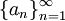# Introduction to Sigma Notation

Previous: Sigma Notation

Next: Sigma Notation Terminology

In many areas of mathematics, we are given an infinite sequenceand we need to add its elements togetherwhich would produce what we will refer to as an infinite sum or infinite series. In producing this infinite sum, we are led to the following initial questions:

• Is there a convenient notation that we can use to represent an infinite sum?
• What properties would the sum have?
• Can we find equivalent representations of the sum?

The last question is of particular importance when

• applying formulas to infinite sums, and
• performing algebraic manipulations upon equations with multiple sums,

as we will see later in the module.

Previous: Sigma Notation

Next: Sigma Notation Terminology